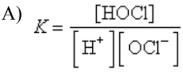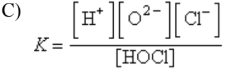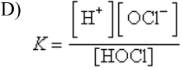# Problem: Which of the following is the equilibrium constant expression for the dissociation of the weak acid HOCl?B) K = [H+][OCl–]E) none of these

###### FREE Expert Solution

For this problem, we have to write the equilibrium constant expression for the dissociation of the weak acid HOCl

Weak acids react with water forming H3O+ and its conjugate base in equilibrium. For HOCl:

91% (417 ratings)###### Problem Details

Which of the following is the equilibrium constant expression for the dissociation of the weak acid HOCl?B) K = [H+][OCl]E) none of these

Frequently Asked Questions

What scientific concept do you need to know in order to solve this problem?

Our tutors have indicated that to solve this problem you will need to apply the Weak Acids concept. If you need more Weak Acids practice, you can also practice Weak Acids practice problems.

What is the difficulty of this problem?

Our tutors rated the difficulty ofWhich of the following is the equilibrium constant expressio...as low difficulty.

What professor is this problem relevant for?

Based on our data, we think this problem is relevant for Professor Rudell's class at San Diego Mesa College.